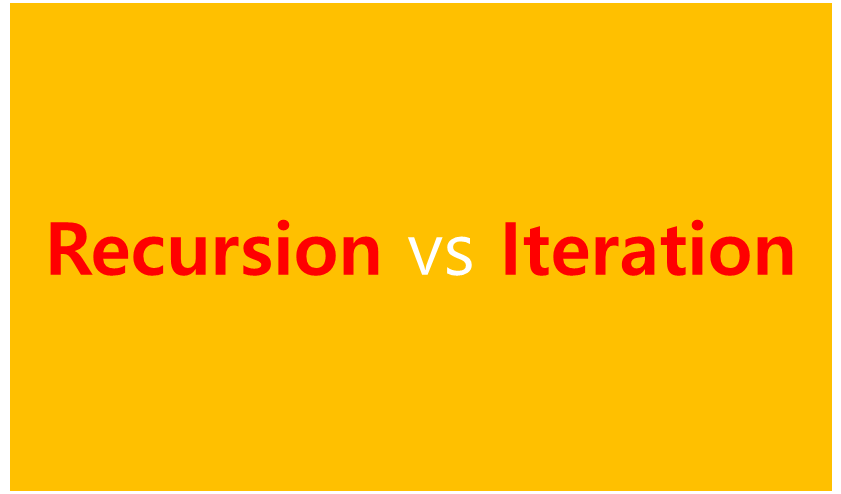# Recursion vs Iteration, What's the difference?

limelimejiwon·2022년 3월 18일
0

## Python으로 공부하는 알고리즘

목록 보기
1/2While I was solving the Binary Search problem, I learned that there is TWO ways to implement "Binary Search", which is Recursive and Iterative approach.

As now, I know that analyzing the complexity of the algorithm is important, I was curious what's the difference between the two and which is more effective.

So, What's the difference between Recursion and Iteration?

Programs are structured to operate repetitive works.

# Difference between Recursion & Iteration

## 1. ⏲ Time Complexity

Yes, this was the first thing I was curious about.

Basically, finding out the Time Complexity of the Recursion is more complicating. Why?

### 🔁 Recursion

• It uses "Recurrence Relation".

❓ What is Recurrence Relation?
an equation that describes a sequence where any term is defined in terms of its previous terms.

• The main point of Recursion is solving the problem using the solution of smaller subproblems.
- If the TC funcion of the algorithm is T(n), then TC of the smaller sub-problems will be defined by the same function but in terms of the input size of the subproblems.
• T(n) = T(input size of subproblem 1) + T(input size of subproblem 2) + ... + T(input size of subproblem k) + TC of additional operations other than recursive calls
• Very high(generally exponential) time complexity.

### 🖐 Iteration

Time Complexity will be defined by the number of cycles being repeated inside the loop.

## ❓ Then, Is Recursion ever faster than looping?

You can find this question-answer in stackoverflow.

It depends on the code and implementation.
For Functional language: Recursion might be faster
For Imperative language: Iteration probably faster
...

I think it is important to understand the inner workings of both in order to choose wisely. I should write new posting with this topic.

## 2. 📝 Usage

We can choose which to use either recursion or iteration, considering Time Complexity and size of the code.

• If the Time Complexity is important and the number of recursive calls would be large 👉 better to use Iteration.
• If the shortness of the code is the issue rather than the Time Complexity 👉 better to use Recurtion.

### 🔁 Recursion

• Since Recursion involves calling the same function again, it has a very small length of code.
• Recursion function is more understandable to read.
• However, the Time Complexity can get to be exponential when there are considerable number of recursive calls.

### 🖐 Iteration

• Iteration is repetition of a block of code. So it involves larger size of the code.
• But the time complexity is generally lesser than it is for recursion.

## Example code - Factorial

# ---- Recursion -----
def factorialUsingRecursion(n):
if n==0:
return 1
return n*factorialUsingRecursion(n-1)
# ---- Iteration -----
def factorialUsingIteration(n):
res=1
for i in range(2,n+1):
res*=i
return res

If you see above code, you can see Recursion is more short and easier to read. On the other hand, Iteration includes more or new variables and it is longer.

## 3. 🧺 Overhead and Infinite Repetition

It's the resource(most often memory and CPU time) required to set up an operation.

1. Recursion has fatal disadvantage in Overhead issue. Recursion has a large amount of Overhead as compared to Iteration.
2. Infinite Repetition in recursion can lead to CPU crash but in iteration, it will stop when memory is exhausted.

### 🔁 Recursion

• In Java, C, and Python, Recursion requires the allocation of a new stack frame, which carries the local state of the call, so it is fairly expensive compared to iteration.
• If the recursion is deep, you can easily run out of the call stak space - what is so-called stack overflow.

One opinion from stackoverflow, "use recursion only for cases where you know there's a limit on the depth it can go to".

### 🖐 Iteration

• Iteration does not involve any such overhead.
• If infinite loops occur,(due to mistake in iterator assignment or increment, or in the terminating condition) it may not lead to system errors, but will surely stop program execution any further.

## 💡 Tail call recursion

It is one kind of recursion that may not suffer from at least the stack overflow problem. ! But the compiler/interpreter should support such optimization.

In this case, the recursive call is done as the last thing the function does. In other words, it ends by returning the value of the recursive call. So the big difference between tail & non-tail recursion is whether there is operation in the return statement or not.

Keeping the caller's frame on stack is a waste of memory because there's nothing left to do once the recursive call returns its value.
So, instead of allocating a new frame for the call, we can reuse the existing one.
As a result, compilers can recognize a tail-recursive function and optiize frame allocation.

## Example1 - Factorial

# ---- Recursion -----
def factorialUsingRecursion(n):
if n==0:
return 1
return n*factorialUsingRecursion(n-1)

How can we change above recursion function to tail-recursion function?
The main point is to make the additional operation unnecessary in the recursvie call part.

# ---- Tail-Recursion -----
def factorialUsingRecursion(n, result=1):
if n==0:
return result
else:
return factorial(n-1, n*result)

## Example2 - Summing Numerical Arrays

# ---- Recursion -----
def sumArray(arr):
if not arr:
return 0
return arr[-1] + sumArray(arr[:-1])
# ---- Tail-Recursion -----
def sumArray(arr, res=0):
if not arr:
return res
return sumArray(arr[:-1], arr[-1]+res)

However, not all functions can be tail-optimized. If we have to process the recursive call’s return value in any way, then tail-call optimization is impossible.